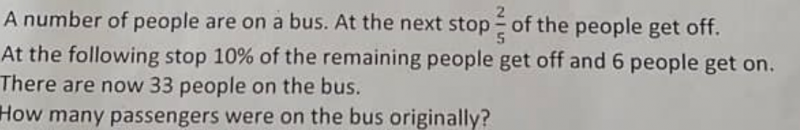# QuestionTotal people on bus before the 6 get on = 33-6 = 27

Fraction of people who got off = 2/5 + (1/10)(3/5) = 23/50         [(1/10)=10%]

Remaining fraction of people on bus = 1- (23/50) = 27/50

Since  27/50  —> 27,  50/50=1 —> 27 x (50/27)  x 1 = 50

Hence the original number of people on the bus was 50

0 Replies 1 Like
```Working:
Suppose there are N people.
1st stop: 3/5 N onboard   (because 2/5 N got off)
2nd stop: 9/10 x 3/5 N + 6 (because 10% of 3/5 N got off and 6 boarded)

So 27/50 N + 6 = 33. That means N = (33-6) x 50/27 = 50.
There were 50 people on the bus initially.

Checking:
start 50
stop1 30 (because 2/5 x 50 = 20 got off)
stop2 27 + 6 = 33 (because 10% x 30 = 3 got off, hence 27; 6 more got on)

```
0 Replies 1 Like